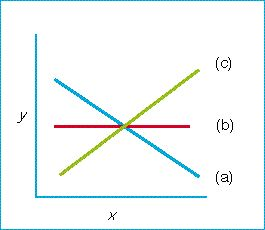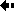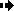## Quantitative genetics - What statistical tools are used by quantitative geneticists?Regression

The regression, symbolized by b xy, between characters X and Y is their covariance divided by the variance of X .

b(x,y) = (cov(x,y))/V(x)

Regressions are used to describe the slopes of graphs and are therefore useful in describing the resemblance between classes of relatives. If X and Y are unrelated, cov xy = 0 and b xy = 0; if they are related, the covariance and regression can be positive or negative.

Figure: relationship between two variables (X and Y). (a) Negative coefficient (b < 0). (b) No relation (b = 0) (c) Positive regression coefficient (b > 0).Previous Next